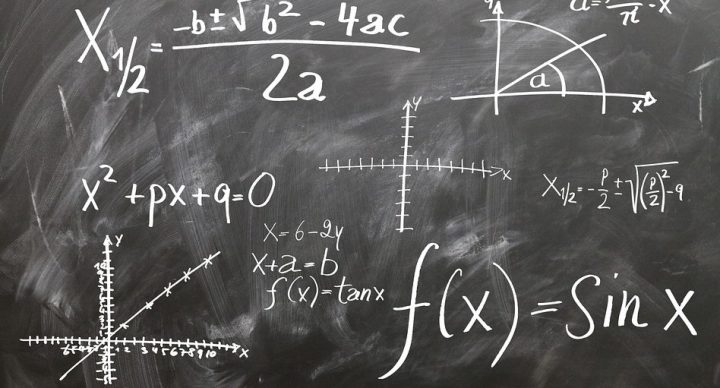# Algebra I – Spanish### Course Overview

Acellus Algebra Spanish teaches students the basic principles, rules, and operations of working with expressions containing variables.  Acellus Algebra Spanish incorporates English math terminology so that Spanish-speaking students can transition to math classes taught in English as effortlessly as possible.

Course topics include the following:
• Order of Operations
• Basic Properties of Algebra
• One- and Two-Step Equations
• Multi-Step Equations
• Literal Equations
• Mixtures
• Uniform Motion
• Formulas for the Equation of a Line (Point-Slope and Slope-Intercept forms)
• Equations for Parallel and Perpendicular Lines
• Parabolas
• Inequalities
• Systems of Equations
• Polynomial Operations
• Factoring Polynomials
• Rational Expressions
• The Distance Formula

This course has been developed for students in grades 8 and above. We recommend that students complete Acellus FunMath Spanish prior to taking this course to ensure that they have a strong foundation of basic mathematical principles on which to build.

This NCTM standards-based course is taught by award-winning Acellus Master Teacher, Nancy Arroyo.

### Course Objectives & Student Learning Outcomes

Upon completion of Acellus Algebra, students will be able to solve algebraic problems that include verbal and algebraic expressions, and open sentences; identify algebraic patterns; solve problems using order of operations and basic algebraic properties to include distributive; solve problems with integers, number sets, and absolute value; compare, order, add, subtract, multiply, and divide rational numbers; calculate square roots, squares, and irrationals; solve one-step equations using addition, subtraction, multiplication, and division; find properties of equality; solve two-step equations with manipulatives; solve complement and supplement problems; clear fractions and decimals; solve number problems; solve perimeter and angle problems, multistep equations, and literal equations; solve proportions; find percent and percent increase or decrease, simple interest, and probability and odds; find mixtures; and solve uniform motion problems.

Students will also be able to graph linear equations and ordered pairs; write equations from relations; define slope; calculate slope; solve problems using point-slope formula and slope-intercept formula; implement graphing shortcuts; find parallel and perpendicular slopes; solve equations of parallel and perpendicular lines; find midpoints; graph absolute value; solve parabolas problems; solve equations with a calculator; solve inequalities using addition, subtraction, multiplication, and division; solve multi-step inequalities; graph inequalities; solve systems of equations by graphing, substitution, addition and multiplication; compare and choose graphing systems of inequalities; identify and interpret polynomial terminology; add, subtract, multiply, and divide polynomials; and factor polynomials.

Finally, students will be able to simplify rational expressions; divide polynomial by a binomial; multiply, divide, add, and subtract rational expressions; simplify square roots; radical expressions; rationalize denominators; solve radical expressions and equations; solve problems using the distance formula; solve quadratic equations; complete the square; solve problems with the rules of exponents; convert units for derived quantities; add and subtract quantities in different units; average data with different units; convert between measurement systems; solve piece-wise and exponential function problems; model using exponential functions; calculate rate of change; solve problems with linear recursive and non-linear recursive formulas; model; calculate probability; read a two-way table; solve problems with conditional probability, law of large numbers, Pascal’s Triangle, rule of four, and quadratic equations; and analyze situations including systems of equations and systems of inequalities.

### Scope and SequenceThis course was developed by the International Academy of Science. Learn More